# List of Medical Abbreviations (A to Z)

List of Medical Abbreviations (A to Z)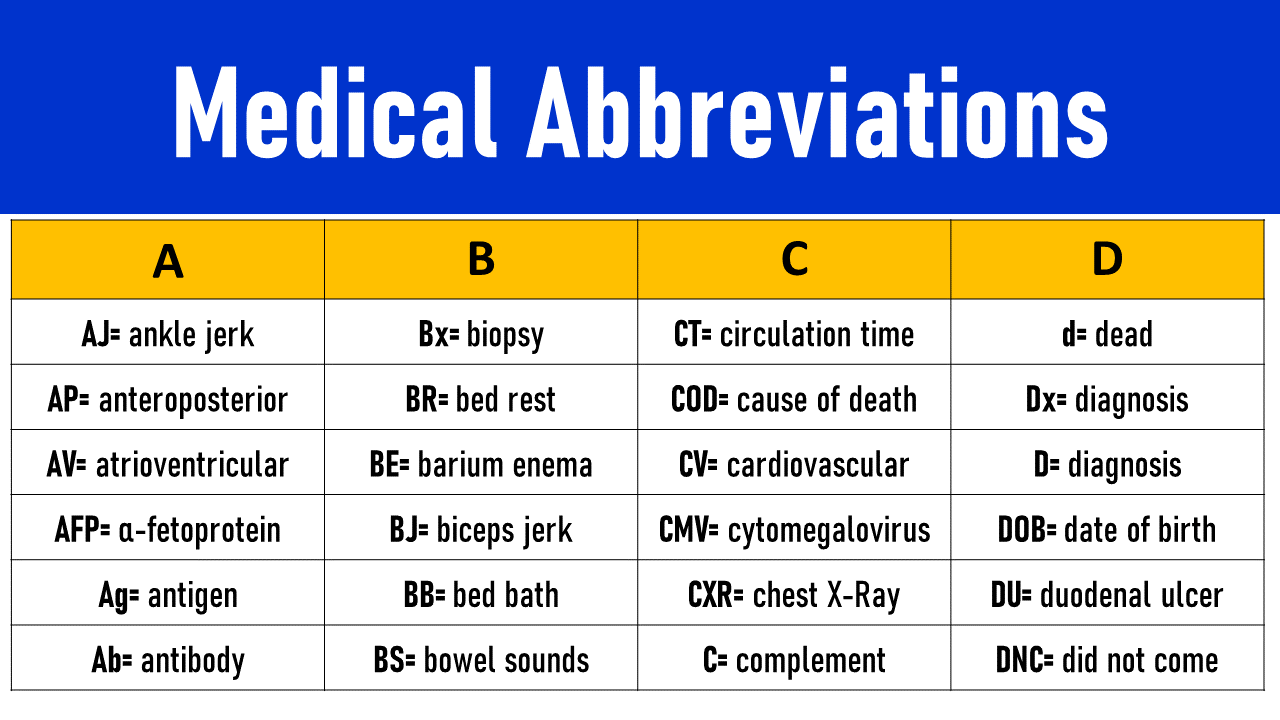Medical abbreviations are commonly used in the English language to shorten long phrases and words. While there are many medical abbreviations, some of the more common ones include AIDS, ADHD, and OCD. By using medical abbreviations, doctors are able to communicate more effectively with one another, as well as with their patients. In addition, medical abbreviations can help to save time during a doctor’s visit.

## Medical Abbreviations List

### Medical Abbreviation – A

• AJ= ankle jerk
• Abd= abdomen
• ABE= acute bacterial endocarditis
• AKA= above knee amputation
• ANA= antinuclear antibody
• ADHD= attention deficit hyperactivity disorder
• AP= anteroposterior
• ADL= activities of daily living
• AST= aspartate transaminase
• AGL= acute granulocytic leukaemia
• APPT= activated partial thromboplastin time
• AV= atrioventricular
• A & W= alive and well
• ASD= atrial septal defect
• AL= acute leukaemia
• AI= aortic incompetence
• AHD= autoimmune haemolytic disease
• APSAC= anisoylated plasminogen
• ABR= absolute bed rest
• AS= anal sphincter
• A & E= accident and emergency
• AED= antiepileptic drug
• A= atrial fibrillation
• ALL= acute lymphocytic leukaemia
• ARDS= adult respiratory distress syndrome
• AFP= α-fetoprotein
• AAA= abdominal aortic aneurysm
• AGN= acute glomerular nephritis
• ABG= arterial blood gases
• ALT= alanine transaminase
• ATN= acute tubular necrosis
• AOB= alcohol on breath
• Ag= antigen
• ASB= asymptomatic bacteriuria
• AB= apex beat
• ARF= acute renal failure
• Ab= antibody
• ASAP= as soon as possible
• AIDS= acquired immune deficiency syndrome
• AFB= acid-fast bacillus
• ACE= angiotensin-converting enzyme
• AMI= acute myocardial infarction### Medical Abbreviation – B

• Bx= biopsy
• BR= bed rest
• BKA= below knee amputation
• BMR= basal metabolic rate
• BE= barium enema
• BCC= basal cell carcinoma
• BBA= born before arrival
• BBT= basal body temperature
• BBB= blood-brain barrier
• BJ= biceps jerk
• BM= bone marrow
• BNO= bowels not open
• BMI= body mass index
• BP= blood pressure
• BB= bed bath
• BPH= benign prostatic hypertrophy
• BS= bowel sounds
• BaE= barium enema
• BOR= bowels open regularly
• BCG= bacille Calmette-Guerin
• BT= bed time
• BUN= blood urea nitrogen
• BWO= bowels well open
• BSA= body surface area
• BW= body water### Medical Abbreviation – C

• CVA= cerebrovascular accident
• CPK= creatine phosphokinase
• COAD= chronic obstructive airways disease
• CHD= coronary heart disease
• CGN= chronic glomerular nephritis
• CT= circulation time
• CGL= chronic granulocytic leukaemia
• CHF= congestive heart failure
• CPAP= continuous positive airway pressure
• CRD= chronic renal disease
• COD= cause of death
• CV= cardiovascular
• CHB= complete heart block
• CVP= central venous pressure
• CEA= carcinoembryonic antigen
• CRF= chronic renal failure
• CAT= computer assisted tomography
• CCF= congestive cardiac failure
• CMV= cytomegalovirus
• CXR= chest X-Ray
• CABG= coronary artery bypass graft
• CAVHD= continuous arteriovenous haemodialysis
• CF= complement fixation
• CBC= complete blood count
• C= complement
• COPD= chronic obstructive pulmonary disease
• CFT= complement fixation test
• CL= clubbing
• CLL= chronic lymphocytic leukaemia
• CK= creatine kinase
• CPR= cardiopulmonary resuscitation
• CHP= charcoal haemoperfusion
• CML= chronic myelocytic leukaemia
• CSF= cerebrospinal fluid
• CSM= cerebrospinal meningitis
• CICr= creatinine clearance
• Cy= cyanosis
• CVD= cardiovascular disease
• CC= chief complaint
• CSU= catheter specimen of urine
• CPD= continuous peritoneal dialysis
• CI= cardiac index
• CRP= C-reactive protein
• Cx= cervix
• CVS= cardiovascular system
• CO= cardiac output
• C & S= culture and sensitivity
• C & P= cystoscopy and pyelogram
• CAPD= continuous ambulatory peritoneal dialysis
• CBS= chronic brain syndrome
• CCU= coronary care unit
• CNS= central nervous system
• COLD= chronic obstructive lung disease
• CA= cancer
• c/o= complains of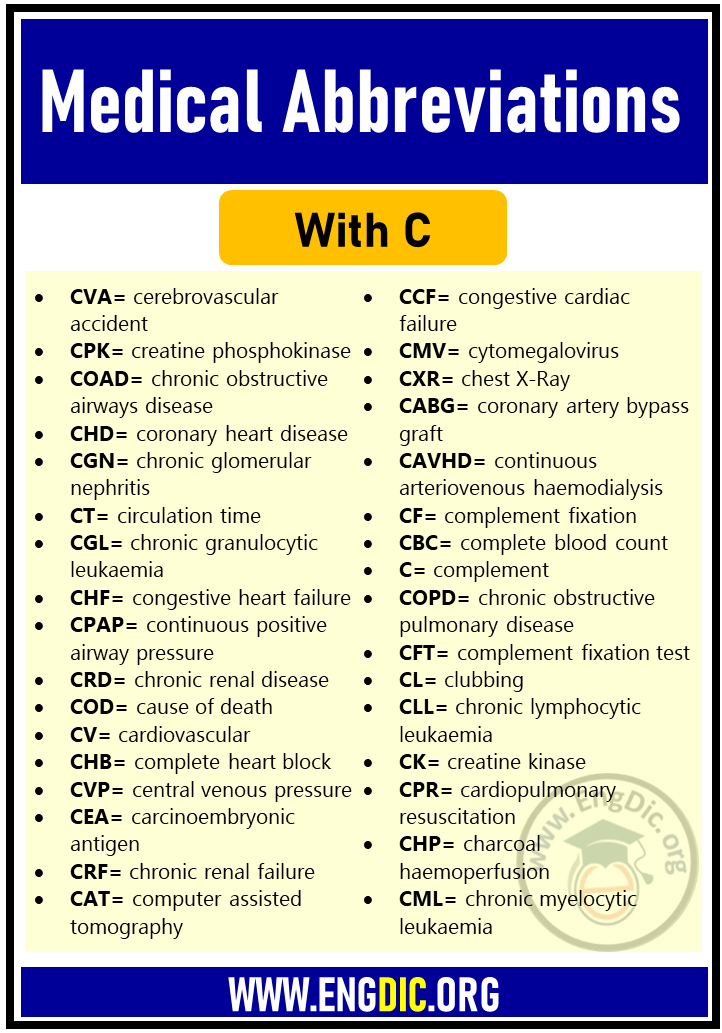### Medical Abbreviation – D

• D & E= dilation and evacuation
• DJD= degenerative joint disease
• DM= diabetes mellitus
• Dx= diagnosis
• DTNT= door to needle time
• DBP= diastolic blood pressure
• DST= dexamethasone suppression test
• DNA= did not attend (outpatients)
• D & C= dilatation and curettage
• DNC= did not come
• D/W= discussed with
• DH= drug history
• DUB= dysfunctional uterine bleeding
• DU= duodenal ulcer
• DI= drug information
• DDU= drug dependency unit
• DVT= deep vein thrombosis
• DOE= dyspnoea on exertion
• DT= delirium tremens
• DNR= do not resuscitate
• D & V= diarrhoea and vomiting
• DOB= date of birth
• D= diagnosis
• DIC= disseminated intravascular coagulation
• DKA= diabetic ketoacidosis
• DXT= deep X-ray therapy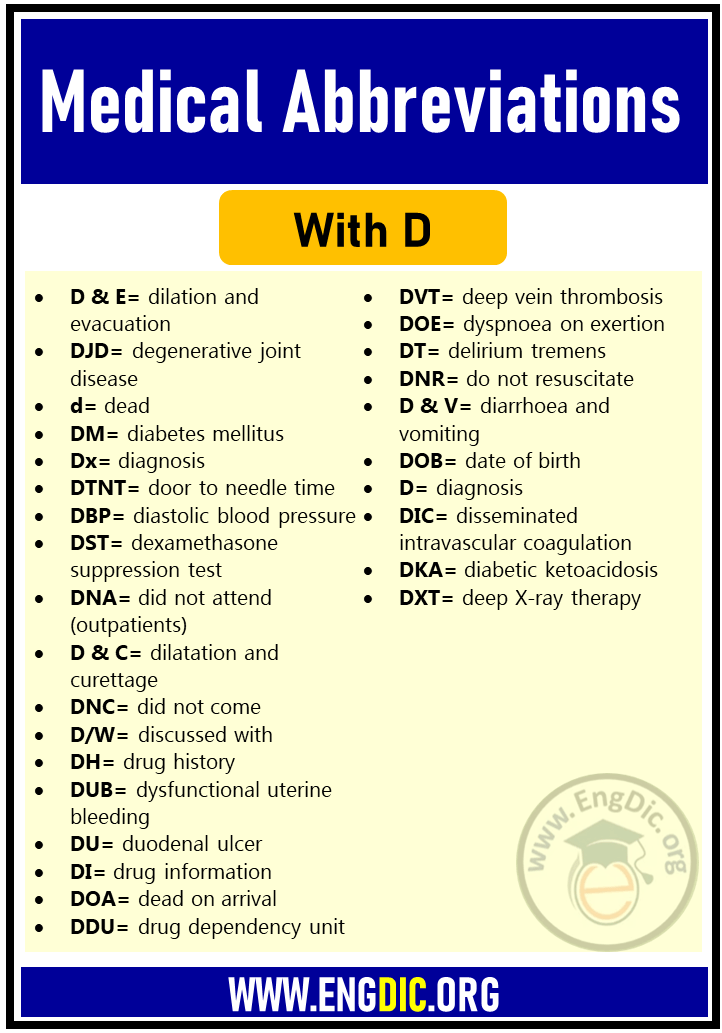### Medical Abbreviation – E

• ESN= educationally subnormal
• ENT= ear, nose and throat
• EEG= electroencephalogram
• EENT= eyes, ears, nose and throat
• EBV= Epstein-Barr virus
• ESRF= end stage renal failure
• ECT= electroconvulsive therapy
• EPO= erythropoietin
• EMG= electromyogram
• ESP= end-systolic pressure
• ESR= erythrocyte sedimentation rate
• EP= ectopic pregnancy
• ECHO= echocardiogram
• ECF= extracellular fluid
• EDD= expected date of delivery
• ESM= ejection systolic murmur
• EBM= expressed breast milk
• ECG= electrocardiogram
• EM= ejection murmur
• ELBW= extremely low birth weight
• ERPC= evacuation of retained products of conception
• ET= endotracheal tube
• EFA= essential fatty acids
• EtOH= ethyl alcohol### Medical Abbreviation – F

• FHR= foetal heart rate
• FHS= foetal heart sounds
• FT4= free thyroxine
• FVC= forced vital capacity
• Fx.= fracture
• FEV 1= forced expiratory volume in 1 second
• FOB= faecal occult blood
• FSH= follicle-stimulating hormone
• FBS= fasting blood sugar
• FBC= full blood count
• FFA= free fatty acids
• FLP= fasting lipid profile
• FUO= fever of unknown origin
• FFP= fresh frozen plasma
• FH= family history
• FP= family planning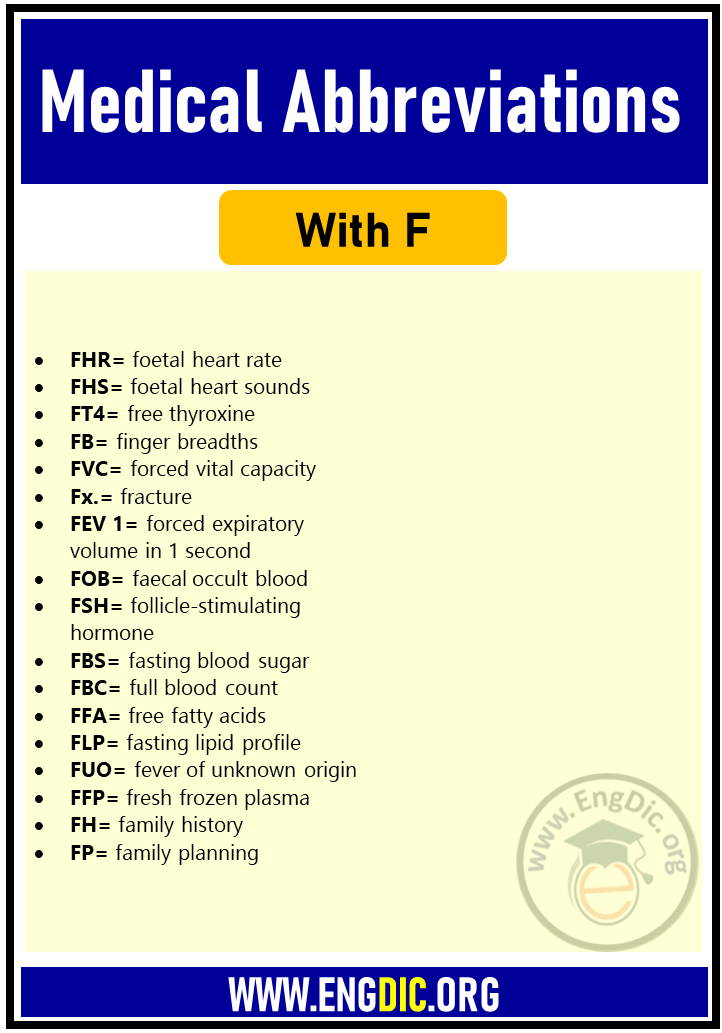### Medical Abbreviation – G

• GI(T)= gastrointestinal (tract)
• GA= general anaesthesia
• G-CSF= granulocyte colony-stimulating factor
• grav.= gravid (pregnant)
• g-GT= γ-glutamyl transferase
• GFR= glomerular filtration rate
• GU= gastric ulcer
• GABA= γ-aminobutyric Acid
• GS= general surgery
• GC= gonococcal
• GE= gastroenterology
• GTT= glucose tolerance test
• GCS= glasgow coma scale
• GH= growth hormone
• GUS= genitourinary system
• Gyn.= gynaecology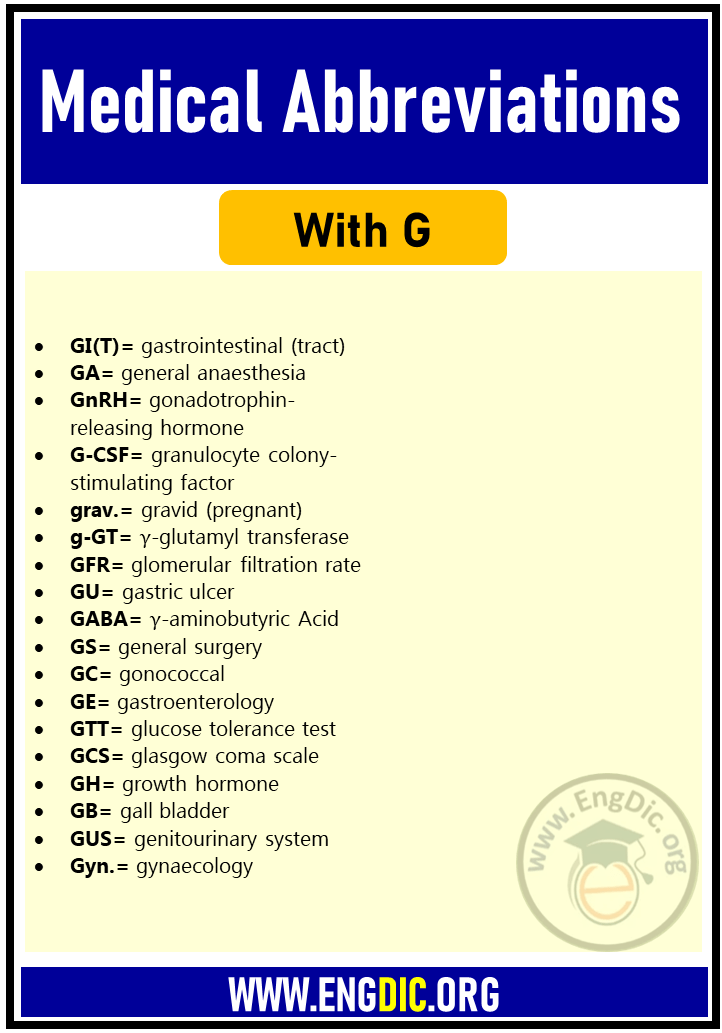### Medical Abbreviation – H

• HS= heart sounds
• HIV= human immunodeficiency virus
• HF= heart failure
• HDU= high dependency unit
• H & L= heart and lungs
• HBP= high blood pressure
• HA= haemolytic anaemia
• H & P= history and physical
• HCVD= hypertensive cardiovascular disease
• HBF= hepatic blood flow
• HDL= high-density lipoprotein
• HD= heart disease
• h/o or H/O= history of
• HHV= human herpes virus
• HLA= human leukocyte antigen
• HHD= hypertensive heart disease
• HbA1= glycosylated haemoglobin
• HPC= history of present complaint
• HB= heart block
• HMMA= 4-hydroxy-3-methoxymandelic acid
• HR= heart rate
• HCV= hepatitis C virus
• HBV= hepatitis B virus
• HRT= hormone replacement therapy
• H= hypodermic
• HSV= herpes simplex virus
• Hct.= haematocrit
• HbF= foetal haemoglobin
• HPV= human papilloma virus
• hep= hepatitis
• Hb= haemoglobin
• HT= hypertension
• HTVD= hypertensive vascular disease
• HBD= hydroxybutyrate dehydrogenase
• HVA= homovanillic acid
• HSVE= herpes simplex virus encephalitis
• HUS= haemolytic uraemic syndrome
• Hx.= history### Medical Abbreviation – I

• IVSD= interventricular septal defect
• IPPB= intermittent positive-pressure breathing
• IOP= intraocular pressure
• INR= international normalised ratio
• IPPV= intermittent positive pressure ventilation
• IVU= intravenous urography
• IP= intraperitoneal
• IM= infectious mononucleosis
• ICU= intensive care unit
• Ig= immunoglobulin
• IUP= intrauterine pregnancy
• ICF= intracellular fluid
• ID= initial dose
• imp= impression
• IDDM= insulin-dependent diabetes mellitus
• IA= intra-amniotic
• inf= inferior
• ITT= insulin tolerance test
• IU= international unit
• ITP= idiopathic thrombocytopenic purpura
• ISA= intrinsic sympathomimetic activity
• IUD= intrauterine device
• ITU= intensive therapy unit
• IF= inspiratory force
• ICM= intercostal margin
• IT= intrathecal
• IVP= intravenous pyelogram
• IBC= iron binding capacity
• ICS= intercostal space
• IDL= intermediate-density lipoprotein
• IHD= ischaemic heart disease
• IV= intravenous
• IBS= irritable bowel syndrome
• IBD= inflammatory bowel disease### Medical Abbreviation – J

• JVD= jugular venous distention
• JV= jugular vein
• JVP= jugular venous pressure
• J= jaundice

### Medical Abbreviation – K

• KJ= knee jerk
• KVO= keep vein open
• KO= keep open

### Medical Abbreviation – L

• Lap= laparotomy
• LH= left hand
• LMWH= low molecular weight heparin
• LA= left arm
• LDL= low-density lipoprotein
• LOM= limitation of movement
• LVH= left ventricular hypertrophy
• LKS= liver, kidneys, spleen
• LV= left ventricle
• LOPS= length of patient’s stay
• lac= laceration
• LFT= liver function test
• LBBB= left bundle branch block
• LP= lumbar puncture
• LIH= left inguinal hernia
• LD= lethal dose
• LMN= lower motor neurone
• LTC= long-term care
• LLL= left lower lobe
• L & A= light and accommodation
• L & W= living and well
• LDH= lactate dehydrogenase
• LAT or lat= lateral
• LVF= left ventricular failure
• L1, L2 …= lumbar vertebrae 1, 2 …
• L= left
• LSCS= lower segment caesarian section
• LIF= left iliac fossa
• LCM= left costal margin
• LUL= left upper lobe
• LBW= low birth weight
• LE= lupus erythematosus
• LMP= last menstrual period
• LN= lymph node### Medical Abbreviation – M

• MSL= midsternal line
• MM= multiple myeloma
• met= metastasis
• MH= marital history
• MCHC= mean corpuscular haemoglobin concentration
• MAOI= monoamine oxidase inhibitor
• MIC= minimum inhibitory concentration
• MDV= multiple dose vial
• MP= menstrual period
• MLD= minimum lethal dose
• MSU= midstream urine specimen
• MyG= myasthenia gravis
• MND= motor neurone disease
• M1= mitral first sound
• M= male
• MDI= metered-dose inhaler
• MRI= magnetic resonance imaging
• MCT= medium-chain triglycerides
• mEq= milliequivalent
• MS= mitral stenosis
• MCH= mean corpuscular haemoglobin
• MCV= mean corpuscular volume
• ML= midline
• MA= menstrual age
• MI= myocardial infarction
• MRSA= methicillin-resistant Staphylococcus aureus
• MHC= major histocompatibility complex
• MAP= mean arterial pressure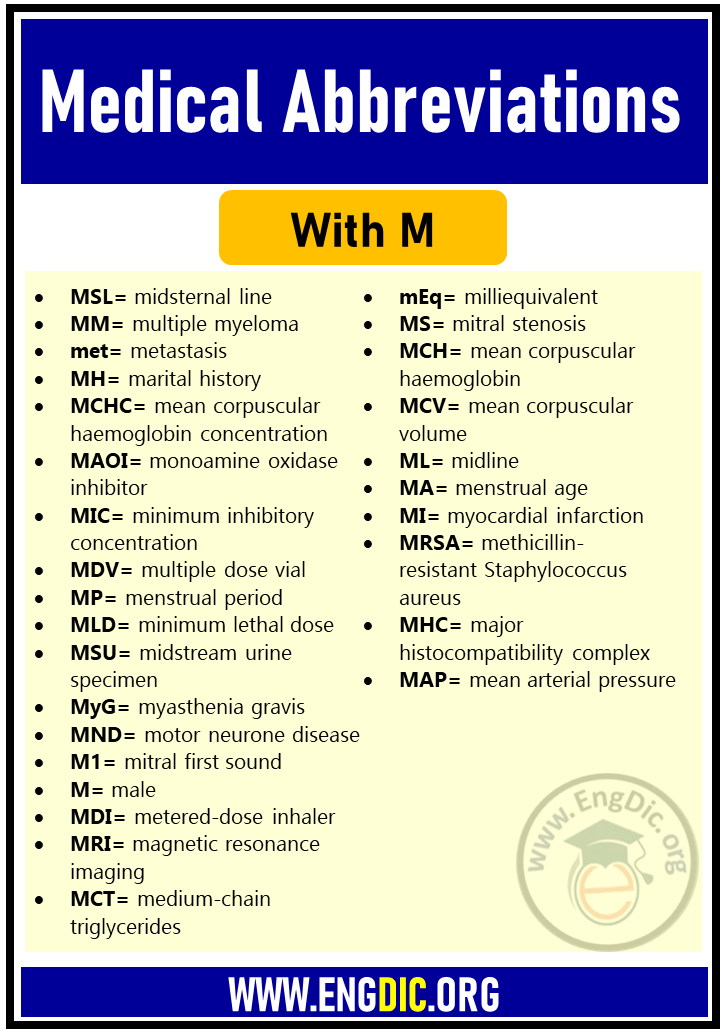### Medical Abbreviation – N

• NKA= no known allergies
• NKHA= non-ketotic hyperosmolar acidosis
• NSAID= non-steroid anti-inflammatory drug
• NOF= neck of femur
• N= normal
• NOK= next of kin
• NS= nervous system
• NVD= nausea, vomiting, diarrhoea
• NG= nasogastric
• NMR= nuclear magnetic resonance
• NNRTI= non-nucleoside reverse transcriptase inhibitor
• NBM= nil by mouth
• NSD= no significant defect
• NARTI= nucleoside analogue reverse transcriptase inhibitor
• NSR= normal sinus rhythm
• NHL= non-Hodgkin’s lymphoma
• NYD= not yet diagnosed
• NL= normal
• NOF= fractured neck of femur
• N & T= nose and throat
• NSU= nonspecific urethritis
• NICU= neonatal intensive care unit
• NIDDM= non-insulin dependent diabetes mellitus
• N & V= nausea and vomiting
• NSFTD= normal spontaneous full-term delivery
• NMS= neuroleptic malignant syndrome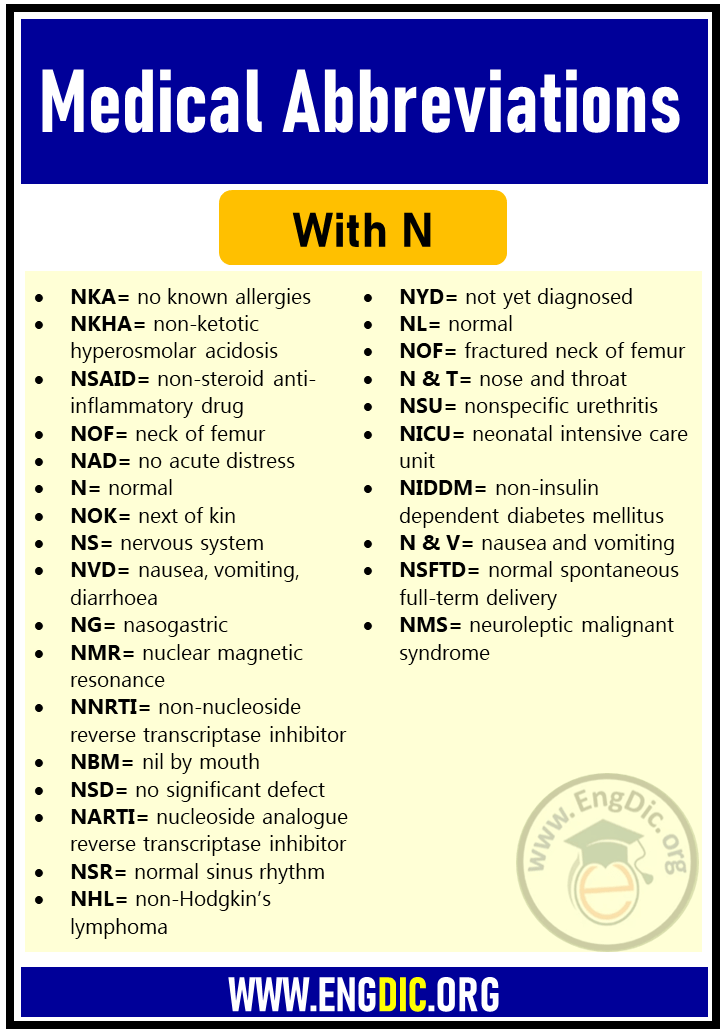### Medical Abbreviation – O

• O/E= on examination
• OGD= oesophogastroduodenoscopy
• OH= occupational history
• OPD= outpatient department
• OP= outpatient
• OA= osteoarthritis
• & A= observation & assessment
• O= oedema
• OC= oral contraceptive
• OPA= outpatient appointment
• OT= occupational therapy
• OB= occult blood
• OR= operating room
• Obs= obstetrics
• OGTT= oral glucose tolerance test
• OU= both eyes
• OS= left eye
• OTC= over the counter
• & E= observation and examination
• OD= overdose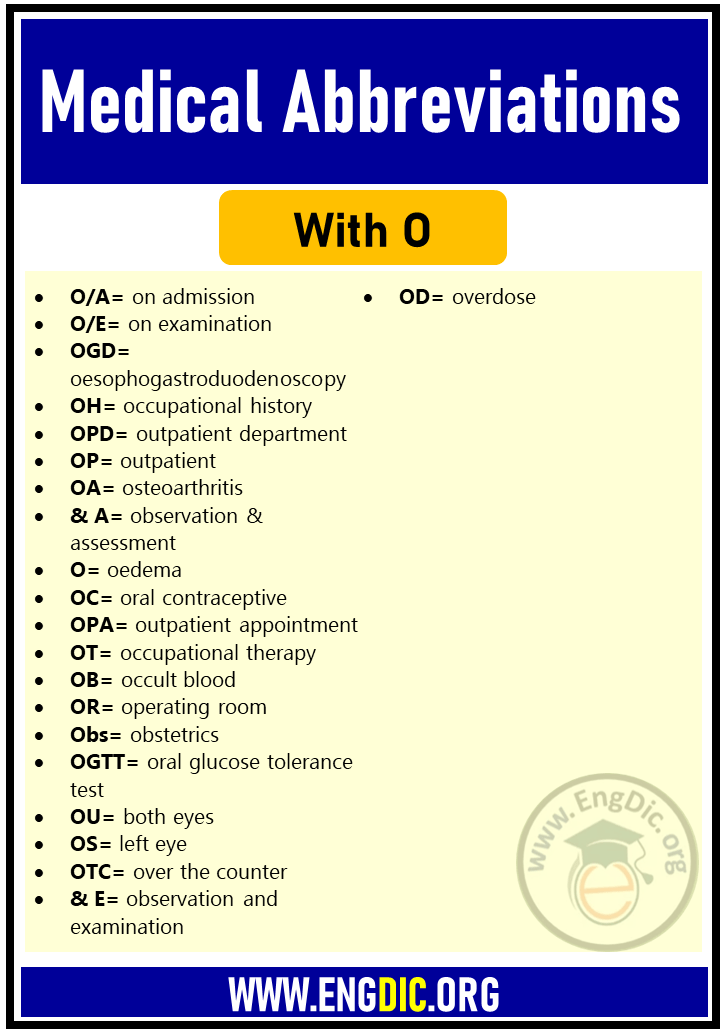### Medical Abbreviation – P

• PMH= past medical history
• pHa= arterial blood pH
• PEFR= peak expiratory flow rate
• PET= positron emission tomography
• PCV= packed cell volume
• PTTK= partial thromboplastin time kaolin
• PTR= prothrombin ratio
• PTH= parathyroid hormone
• PTU= propylthiouracil
• PCP= Pneumocystis cariniipneumonia
• P & A= percussion and auscultation
• PTT= partial thromboplastin time
• PA= pernicious anaemia
• Pt= patient
• PBI= protein bound iodine
• PO= by mouth
• PR= per rectum
• P= pulse
• PVT= paroxysmal ventricular tachycardia
• PN= percussion note
• PCA= patient-controlled analgesia
• PPH= post partum haemorrhage
• PT= physical therapy
• PC= present complaint packed cells
• PPI= proton pump inhibitor
• PMI= past medical illness
• PEEP= positive end-expiratory pressure
• PTA= plasma thromboplastin antecedent
• PUVA= psoralen and ultraviolet A radiation
• PEG= percutaneous endoscopic gastrostomy
• PE= physical examination
• PVC= premature ventricular contraction
• PUO= pyrexia of unknown origin
• PU= peptic ulcer
• PF= peak flow
• PVD= peripheral vascular disease
• PV= per vagina
• PERRLA= pupils equal, round, react to light and accommodation
• PFT= pulmonary function test
• PAWP= pulmonary arterial wedge pressure
• PPTK= partial thromboplastin time kaolin
• P & R= pulse and respiration
• PH= past history
• PND= paroxysmal nocturnal dyspnoea
• PUD= peptic ulcer disease
• PMR= patient medical record
• PMN= polymorphonuclear leucocyte
• PI= present illness
• Pnx= pneumothorax
• ppm= parts per million
• PAT= paroxysmal atrial tachycardia
• PFR= peak flow rate
• PCS= portocaval shunt
• PSA= prostate-specific antigen
• PID= pelvic inflammatory disease
• PKU= phenylketonuria
• Px= past history
• PMB= postmenopausal bleeding
• PICC= peripherally inserted central catheter
• PMT= premenstrual tension
• PMS= postmenopausal syndrome
• PM= post mortem### Medical Abbreviation – R

• RLL= right lower lobe (lung)
• RBS= random blood sugar
• RSV= respiratory syncytial virus
• R= respiration
• RIF= right iliac fossa
• RS= respiratory system
• RBC= red blood cell
• RUL= right upper lobe
• REM= rapid eye movement
• RA= renal artery
• RLD= related living donor
• RSR= regular sinus rhythm
• ROM= range of motion
• RES= reticuloendothelial system
• rt-PA= recombinant tissue-type plasminogen activator
• RV= residual volume
• RR= respiratory rate
• RVH= right ventricular hypertrophy
• RBBB= right bundle branch block
• RDS= respiratory distress syndrome
• RFT= respiratory function test
• Rh factor= rhesus factor
• RR & E= round regular and equal (pupils)
• RHD= rheumatic heart disease
• RBF= renal blood flow
• RF= renal failure
• RQ= respiratory quotient
• RSF= rheumatoid serum factor### Medical Abbreviation – S

• STD= sexually transmitted disease
• SV= stroke volume
• S/B= seen by
• SP= systolic pressure
• SALT= speech and language therapist
• SOA= swelling of ankle(s)
• SIADH= syndrome of inappropriate antidiuretic hormone
• SH= social history
• SB= seen by
• ST= sinus tachycardia
• SBE= subacute bacterial endocarditis
• SL or sl= sublingual
• SIDS= sudden infant death syndrome
• SSS= sick sinus syndrome
• SBP= spontaneous bacterial peritonitis
• SR= sinus rhythm
• stat.= immediately
• SOB= shortness of breath
• SSRI= selective serotonin reuptake inhibitor
• SLE= systemic lupus erythematosus
• SCBU= special care baby unit
• Sx= symptoms
• SI= small intestine
• SBO= small bowel obstruction
• SC= sickle cell
• s= without
• SVT= supraventricular tachycardia
• SA= sinoatrial
• SOAP= subjective, objective, assessment, plan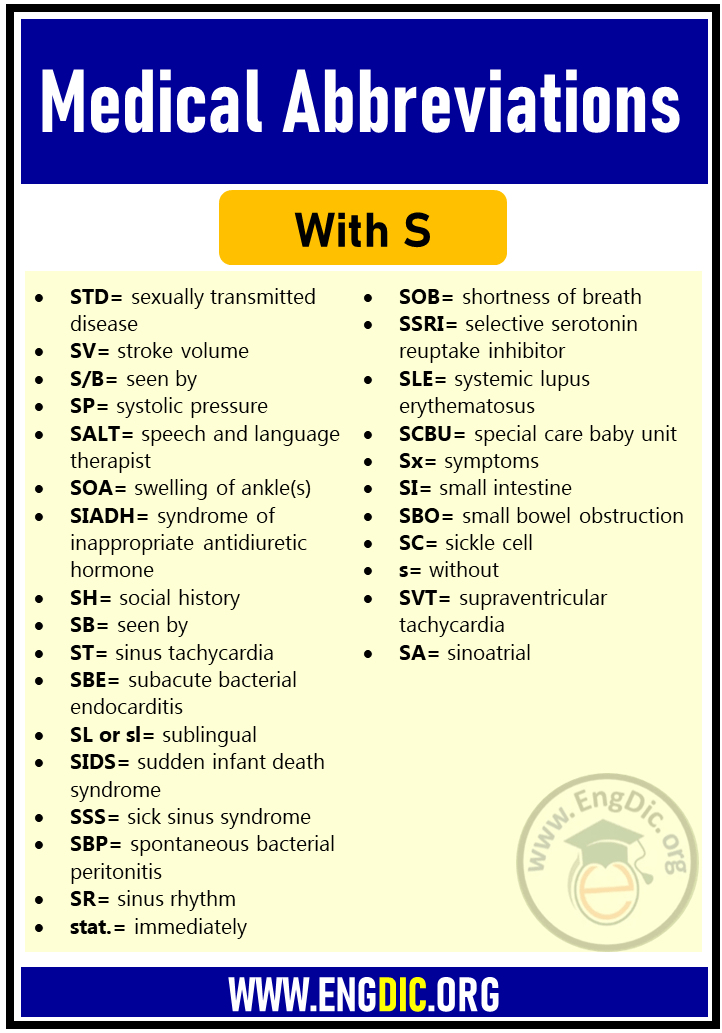### Medical Abbreviation – T

• TL= tubal ligation
• TLC= tender loving care
• TPR= temperature, pulse, respiration
• TBW= total body water
• T3= triiodothyronine
• TOPS= take off pounds sensibly
• TFT= thyroid function test
• TDI= total dose infusion
• t-PA= tissue plasminogen factor
• T= temperature
• TPN= total parenteral nutrition
• TB= tuberculosis
• T & X= type and crossmatch
• TURB= transurethral resection of the bladder
• TCA= tricyclic antidepressant
• TD= tardive dyskinesia
• THR= total hip replacement
• T4= thyroxine
• Tx.= Treatment
• TV= trichomonas vaginalis
• TNF= tumour necrosis factor
• TOP= termination of pregnancy
• TH= thyroid hormone
• TSH= thyroid-stimulating hormone
• TG= triglyceride
• TBS= total body surface
• TTO= to take out
• TIA= transient ischaemic attack
• TURP= transurethral resection of the prostate
• TM= tympanic membrane
• T & A= tonsillectomy and adenoidectomy
• TP= thrombophlebitis
• TBG= thyroid binding globulin
• TDM= therapeutic drug monitoring
• TUR= transurethral resection
• TIBC= total iron-binding capacity
• TKO= to keep open
• TENS= transcutaneous electrical nerve stimulation
• TTA= to take away
• TOF= tracheo-oesophageal fistula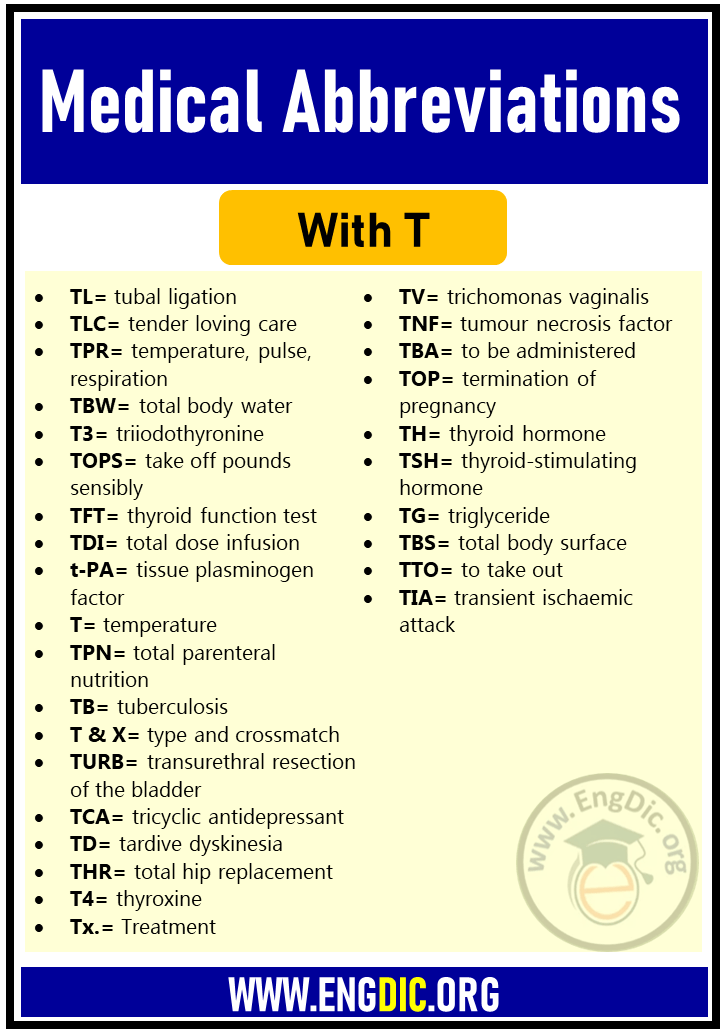### Medical Abbreviation – U

• UA= uric acid
• UC= ulcerative colitis
• UO= urinary output
• URTI= upper respiratory tract infection
• US= ultrasound
• UFH= unfractionated heparin
• UTI= urinary tract infection
• UCO= urinary catheter out
• UDV= unit dose vial
• URI= upper respiratory infection
• U & E= urea & electrolytes
• UK= unknown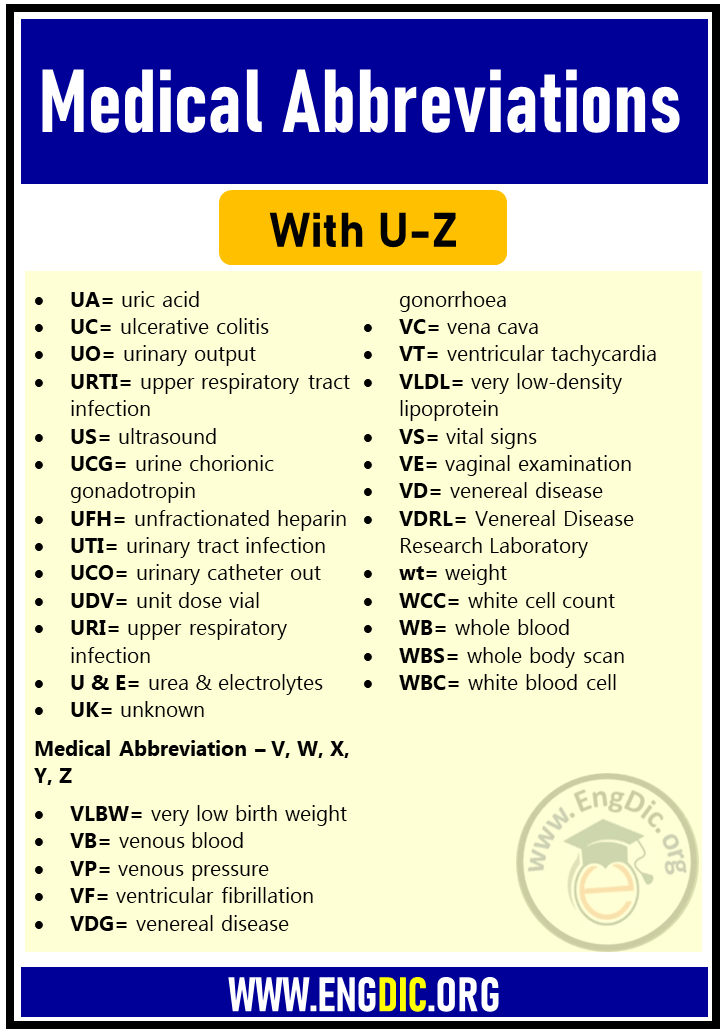### Medical Abbreviation – V, W, X, Y, Z

• VLBW= very low birth weight
• VB= venous blood
• VP= venous pressure
• VF= ventricular fibrillation
• VDG= venereal disease gonorrhoea
• VC= vena cava
• VT= ventricular tachycardia
• VLDL= very low-density lipoprotein
• VS= vital signs
• VE= vaginal examination
• VD= venereal disease
• VDRL= Venereal Disease Research Laboratory
• wt= weight
• WCC= white cell count
• WB= whole blood
• WBS= whole body scan
• WBC= white blood cell
• WPW= Wolff-Parkinson-White syndrome
• XY= male chromosome type
• XX= female chromosome type
• ZES= Zollinger–Ellison syndrome